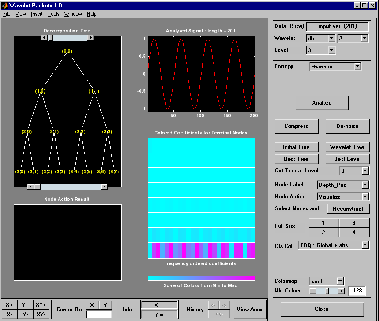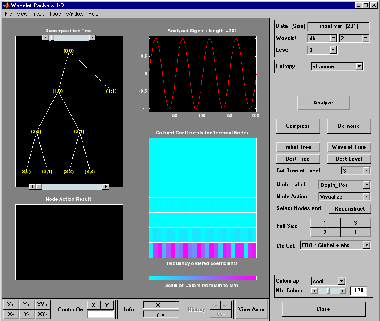# drawtree

Draw wavelet packet decomposition tree (GUI)

## Syntax

```drawtree(T) F = drawtree(T) drawtree(T,F) ```

## Description

`drawtree(T)` draws the wavelet packet tree `T`, and ```F = drawtree(T)``` also returns the figure's handle.

For an existing figure F produced by a previous call to the drawtree function, `drawtree(T,F)` draws the wavelet packet tree T in the figure whose handle is F.

## Examples

```x = sin(8*pi*[0:0.005:1]); t = wpdec(x,3,'db2'); fig = drawtree(t); ``````%--------------------------------------- % Use command line function to modify t. %--------------------------------------- t = wpjoin(t,2); drawtree(t,fig); ```## Version History

Introduced before R2006a